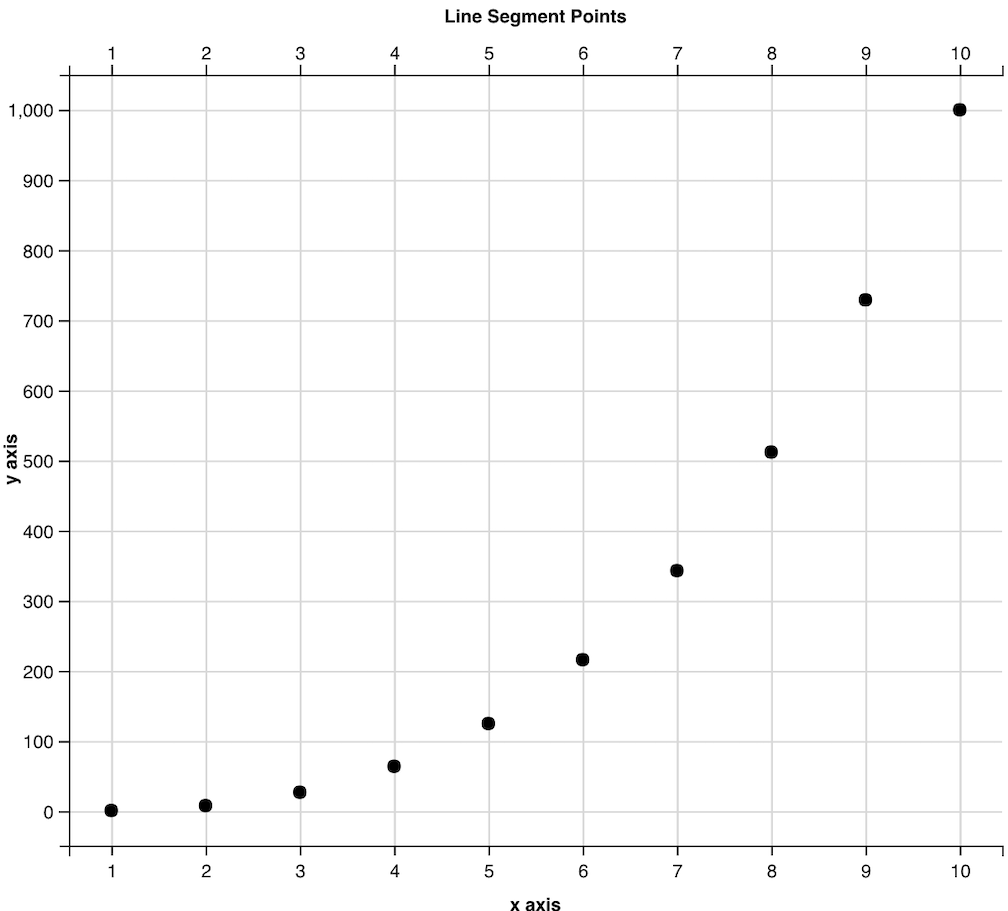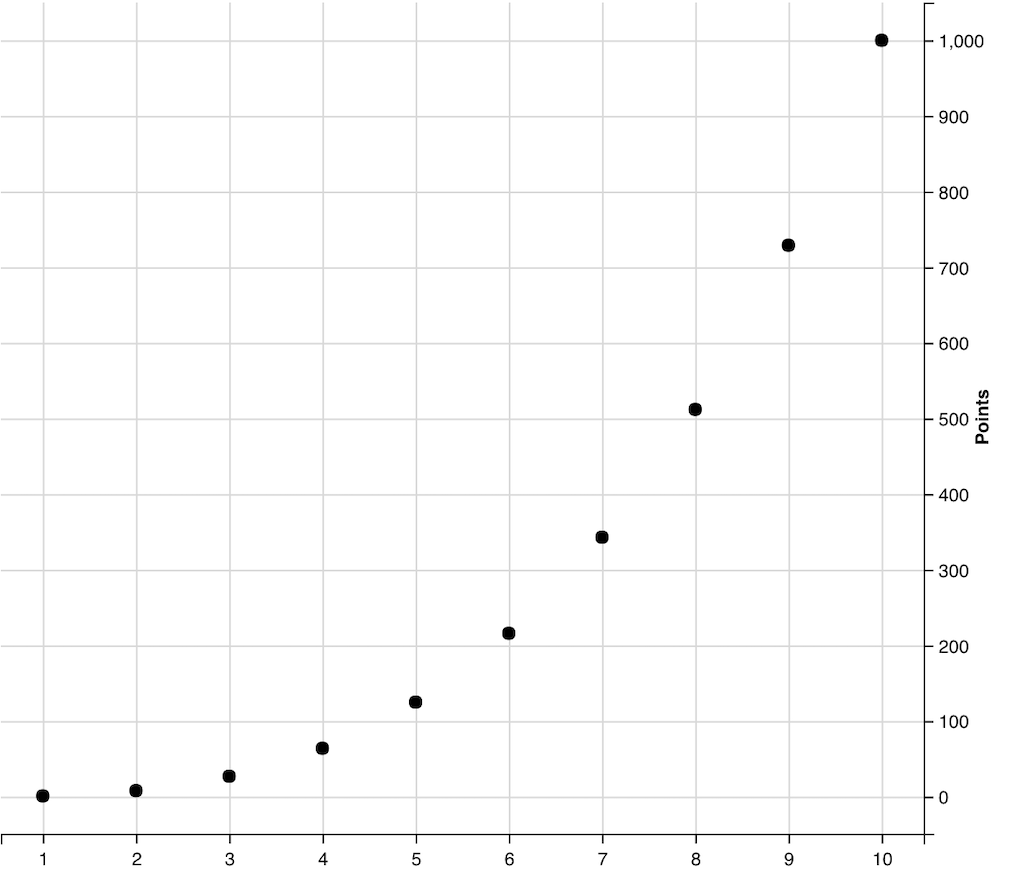Open in App
Not now

# How to Add a plot title to ggvis in R

• Last Updated : 09 Sep, 2022

In this article, we will be looking at the approach to adding a plot title to ggvis package in the R programming language.

The ggvis package in R is used to provide the data visualization. It is used to create visual interactive graphics tools for data plotting and representation. The package can be installed into the working space using the following command :

`install.packages("ggvis")`

The ggvis method in the ggvis package starts the ggvis graphical window. The ggvis method has the following syntax: The ggvis method is used to start ggvis graphical window. The ggvis method has the following syntax :

ggvis( data , mp1, mp2.,)

Arguments :

• data – The dataset to plot
• mp1, mp2,.. – The map variables to plot

The add_axis method is then added to derive the labeling for the axes of the plotted graph. It can be used to override the default value for the axes.

add_axis( vis, axes , title )

Arguments :

• vis – The ggvis object to use for plotting
• axes – The axes to be used for plotting
• title – The title for the axis

Other visualization parameters can also be added, if required, for better readability of the output. For instance, the axis title can be placed at a particular orientation place.

The below code snippet is used to add an axis title to the x and y axes as well as to the graph top along the x direction. The orientation is set to the top with the title “Line Segment Points” .

## R

 `# installing the required libraries` `library``(ggplot2)` `library``(ggvis)`   `# creating the data frame by defining` `# the x and y coordinates respectively` `data_frame < - ``data.frame``(` `    ``x_pos=1: 10,` `    ``y_pos < - x ^ 3` `)` `print``(``"Data Frame"``)` `print``(data_frame)`   `data_frame % > % ``ggvis``(~x_pos, ~y_pos) % > % ``layer_points``() % >%`   `# adding the plot title for x axis` `add_axis``(``"x"``, title=``"x axis"``) % >%`   `# adding the plot title for y axis` `add_axis``(``"y"``, title=``"y axis"``) % >%`   `# adding the plot title on the top of the graph` `add_axis``(``"x"``, orient=``"top"``, title=``"Line Segment Points"``)`

Output

``` "Data Frame"
> print(data_frame)
x_pos y_pos....x.3
1      1            1
2      2            8
3      3           27
4      4           64
5      5          125
6      6          216
7      7          343
8      8          512
9      9          729
10    10         1000```The direction can also be set across the y axis, with the orientation set to right. The default orientation is towards the left along the y axis.

## R

 `# installing the required libraries` `library``(ggplot2)` `library``(ggvis)`   `# creating the data frame by defining` `# the x and y coordinates respectively` `data_frame < - ``data.frame``(` `    ``x_pos=1: 10,` `    ``y_pos < - x ^ 3` `)` `print``(``"Data Frame"``)` `print``(data_frame)`   `data_frame % > % ``ggvis``(~x_pos, ~y_pos) % > % ``layer_points``() % >%`   `# adding the plot title on the right of` `# the graph along the y axis direction` `add_axis``(``"y"``, orient=``"right"``, title=``"Points"``)`

Output

``` "Data Frame"
> print(data_frame)
x_pos y_pos....x.3
1      1            1
2      2            8
3      3           27
4      4           64
5      5          125
6      6          216
7      7          343
8      8          512
9      9          729
10    10         1000```My Personal Notes arrow_drop_up
Related Articles